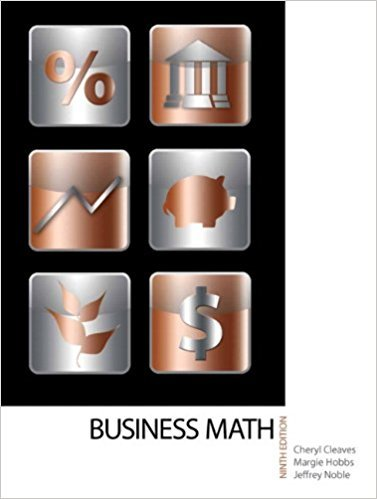×
×

# Subtract. \$52,982.97 - \$45,712.49ISBN: 9780135108178 355

## Solution for problem 9 Chapter 3-2

• Textbook Solutions
• 2901 Step-by-step solutions solved by professors and subject experts
• Get 24/7 help from StudySoup virtual teaching assistants4 5 1 305 Reviews
28
3
Problem 9

Subtract. \$52,982.97 - \$45,712.49

Step-by-Step Solution:
Step 1 of 3

Homework 3.1 and 3.2 Non right triangles require a different method The law of sines: sinα sinβ sinγ = = a b c Five types of problems ASA AAS ASS SAS SSS AAS (angle angle side) α = 37 β = 87 a = 17 All angles still add up to 180 α = 37 o a =17 o 17sin87 β = 87 b = sin37 = 28.2...

Step 2 of 3

Step 3 of 3

##### ISBN: 9780135108178

Unlock Textbook Solution